# 用 Signal Processing Toolbox 软件对数据进行滤波

### 低通 FIR 滤波器 - 加窗方法

```rng default Fs = 1000; t = linspace(0,1,Fs); x = cos(2*pi*100*t)+0.5*randn(size(t));```

```fc = 150; Wn = (2/Fs)*fc; b = fir1(20,Wn,'low',kaiser(21,3)); [h,f] = freqz(b,1,[],Fs); plot(f,mag2db(abs(h))) xlabel('Frequency (Hz)') ylabel('Magnitude (dB)') grid```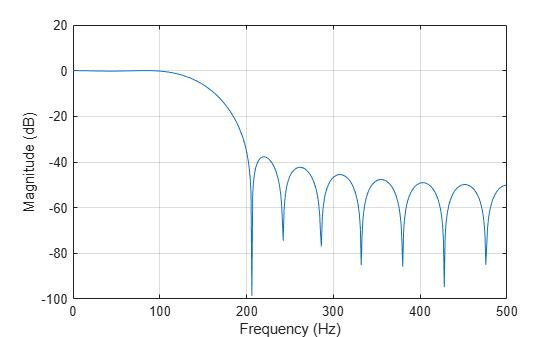```y = filter(b,1,x); plot(t,x,t,y) xlim([0 0.1]) xlabel('Time (s)') ylabel('Amplitude') legend('Original Signal','Filtered Data')```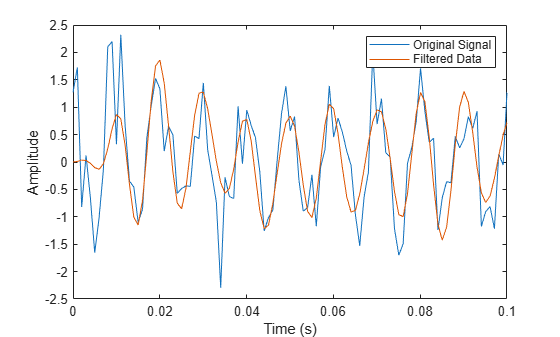```Fs = 1000; Hd = designfilt('lowpassfir','FilterOrder',20,'CutoffFrequency',150, ... 'DesignMethod','window','Window',{@kaiser,3},'SampleRate',Fs);```

```y1 = filter(Hd,x); plot(t,x,t,y1) xlim([0 0.1]) xlabel('Time (s)') ylabel('Amplitude') legend('Original Signal','Filtered Data')```### 使用滤波器设计工具设计低通 FIR 滤波器

• 在命令行中输入 `filterDesigner` 启动滤波器设计工具。

• 响应类型设置为低通

• 设计方法设置为 FIR，并选择窗口方法。

• 滤波器阶数下，选择指定阶数。将阶设置为 20。

• 频率设定下，将单位设置为 Hz，将 Fs 设置为 1000，并将 Fc 设置为 150。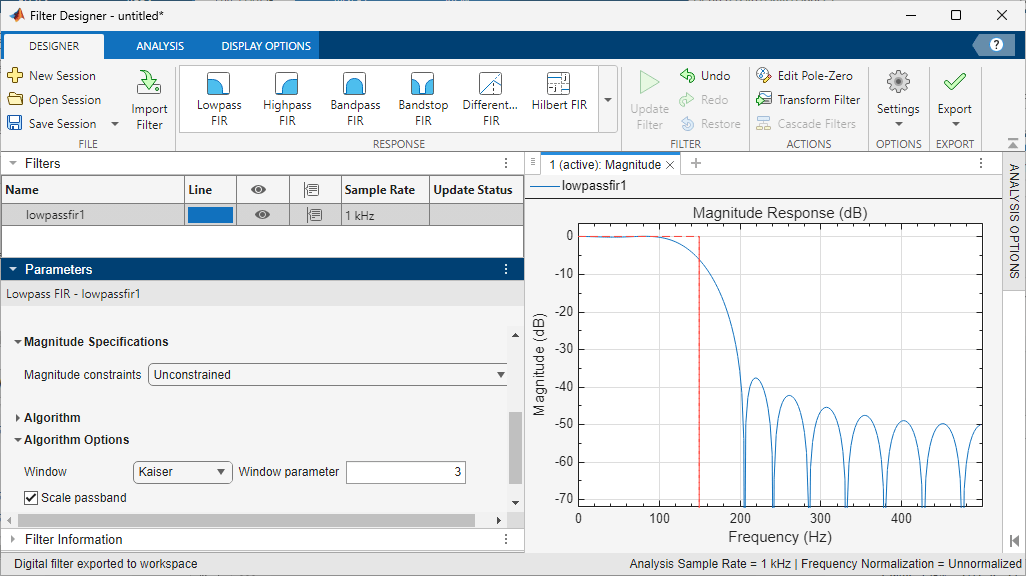• 点击设计滤波器

• 选择文件 > 导出...，将您的 FIR 滤波器作为系数或滤波器对象导出到 MATLAB® 工作区。在本示例中，将滤波器导出为对象。将变量名称指定为 `Hd`• 点击导出

• 用导出的滤波器对象对命令行窗口中的输入信号进行滤波。绘制 100 Hz 正弦波的前十个周期的结果。

```y2 = filter(Hd,x); plot(t,x,t,y2) xlim([0 0.1]) xlabel('Time (s)') ylabel('Amplitude') legend('Original Signal','Filtered Data')```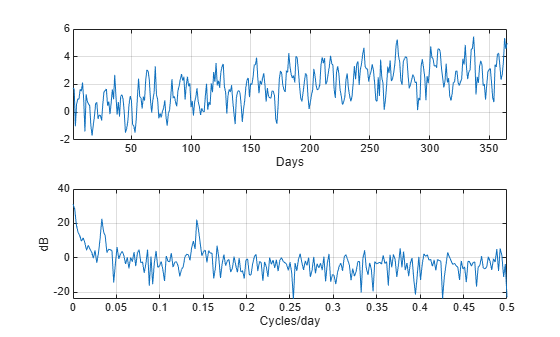• 选择文件 > 生成 MATLAB 代码 > 滤波器设计函数生成使用您的设定创建滤波器对象的 MATLAB 函数。

### 带通滤波器 - 最小阶 FIR 和 IIR 系统

```rng default Fs = 1; n = 1:365; x = cos(2*pi*(1/7)*n)+cos(2*pi*(1/30)*n-pi/4); trend = 3*sin(2*pi*(1/1480)*n); y = x+trend+0.5*randn(size(n)); [pxx,f] = periodogram(y,[],[],Fs); subplot(2,1,1) plot(n,y) xlim([1 365]) xlabel('Days') grid subplot(2,1,2) plot(f,10*log10(pxx)) xlabel('Cycles/day') ylabel('dB') grid```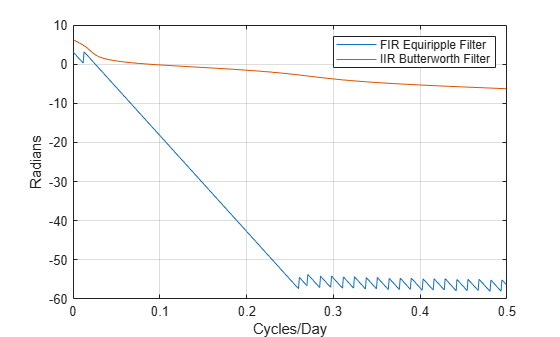```Hd1 = designfilt('bandpassfir', ... 'StopbandFrequency1',1/60,'PassbandFrequency1',1/40, ... 'PassbandFrequency2',1/4 ,'StopbandFrequency2',1/2 , ... 'StopbandAttenuation1',10,'PassbandRipple',1, ... 'StopbandAttenuation2',10,'DesignMethod','equiripple','SampleRate',Fs); Hd2 = designfilt('bandpassiir', ... 'StopbandFrequency1',1/60,'PassbandFrequency1',1/40, ... 'PassbandFrequency2',1/4 ,'StopbandFrequency2',1/2 , ... 'StopbandAttenuation1',10,'PassbandRipple',1, ... 'StopbandAttenuation2',10,'DesignMethod','butter','SampleRate',Fs);```

`fprintf('The order of the FIR filter is %d\n',filtord(Hd1))`
```The order of the FIR filter is 78 ```
`fprintf('The order of the IIR filter is %d\n',filtord(Hd2))`
```The order of the IIR filter is 8 ```
```[phifir,w] = phasez(Hd1,[],1); [phiiir,w] = phasez(Hd2,[],1); figure plot(w,unwrap(phifir)) hold on plot(w,unwrap(phiiir)) hold off xlabel('Cycles/Day') ylabel('Radians') legend('FIR Equiripple Filter','IIR Butterworth Filter') grid```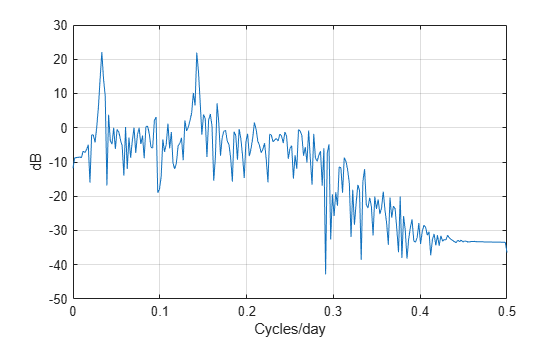IIR 滤波器的阶数比 FIR 滤波器低得多。然而，FIR 滤波器在通带上具有线性相位响应，而 IIR 滤波器则没有。FIR 滤波器以均等的方式延迟滤波器通带中的所有频率，而 IIR 滤波器不延迟。

```Hdlow = designfilt('lowpassfir', ... 'PassbandFrequency',1/4,'StopbandFrequency',1/2, ... 'PassbandRipple',1,'StopbandAttenuation',10, ... 'DesignMethod','equiripple','SampleRate',1);```

```yfir = filter(Hd1,y); yiir = filter(Hd2,y); ylow = filter(Hdlow,y);```

```[pxx,f] = periodogram(yiir,[],[],Fs); plot(f,10*log10(pxx)) xlabel('Cycles/day') ylabel('dB') grid```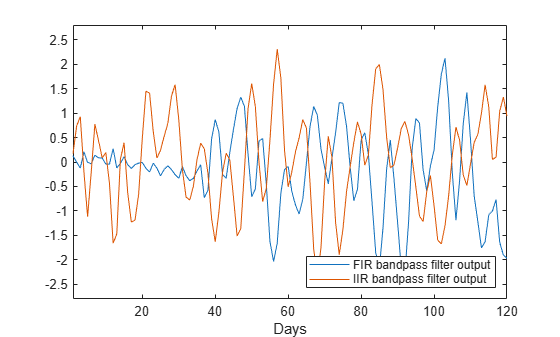PSD 估计值显示带通滤波器使低频趋势和高频噪声发生了衰减。

```plot(n,yfir,n,yiir) axis([1 120 -2.8 2.8]) xlabel('Days') legend('FIR bandpass filter output','IIR bandpass filter output', ... 'Location','SouthEast')```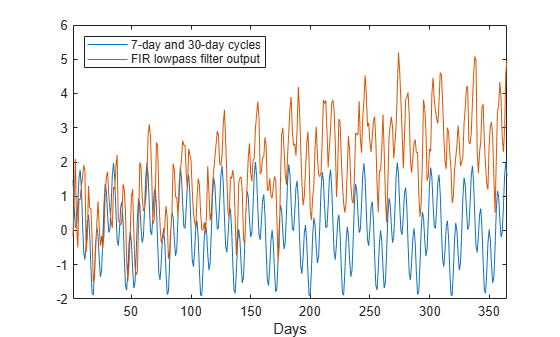FIR 滤波器中增加的相位延迟在滤波器输出中很明显。

```plot(n,x,n,ylow) xlim([1 365]) xlabel('Days') legend('7-day and 30-day cycles','FIR lowpass filter output', ... 'Location','NorthWest')```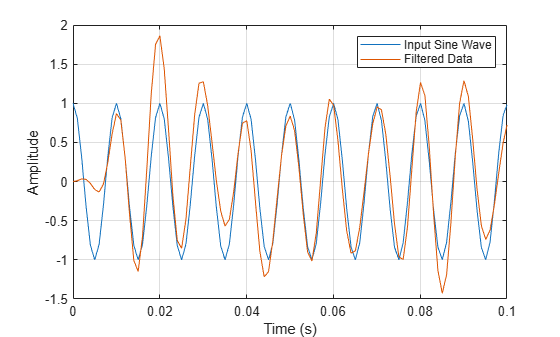### 零相位滤波

```rng default Fs = 1000; t = linspace(0,1,Fs); x = cos(2*pi*100*t)+0.5*randn(size(t)); % Using fir1 fc = 150; Wn = (2/Fs)*fc; b = fir1(20,Wn,'low',kaiser(21,3)); % Using designfilt Hd = designfilt('lowpassfir','FilterOrder',20,'CutoffFrequency',150, ... 'DesignMethod','window','Window',{@kaiser,3},'SampleRate',Fs);```

```yout = filter(Hd,x); xin = cos(2*pi*100*t); plot(t,xin,t,yout) xlim([0 0.1]) xlabel('Time (s)') ylabel('Amplitude') legend('Input Sine Wave','Filtered Data') grid```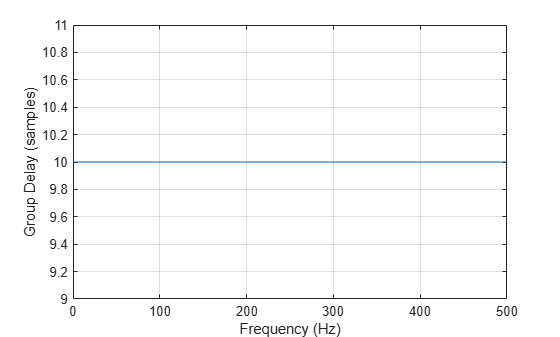```[gd,f] = grpdelay(Hd,[],Fs); plot(f,gd) xlabel('Frequency (Hz)') ylabel('Group Delay (samples)') grid```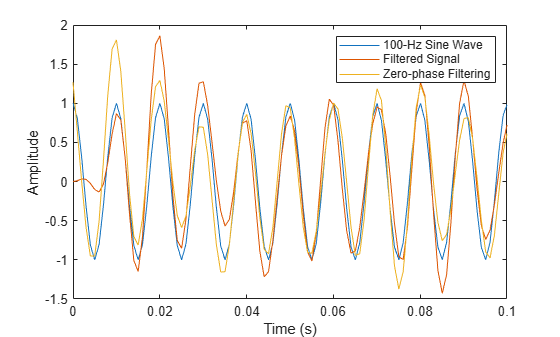```yzp = filtfilt(Hd,x); plot(t,xin,t,yout,t,yzp) xlim([0 0.1]) xlabel('Time (s)') ylabel('Amplitude') legend('100-Hz Sine Wave','Filtered Signal','Zero-phase Filtering',... 'Location','NorthEast')```